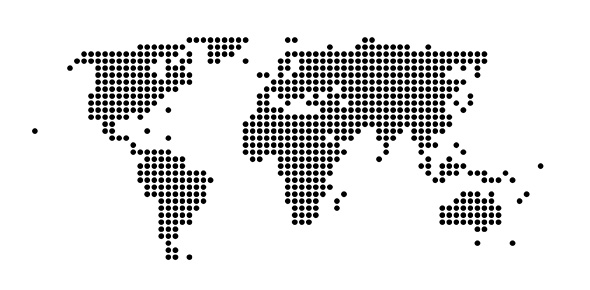# It Officer Scale-1 Test

9 QuestionsSettingsRelated Topics
• 1.
A computer assisted method for the recording and analyzing of existing or hypothetical systems is
• A.

Data transmission

• B.

Data flow

• C.

Data capture

• D.

Data processing

• E.

None of The Above

• 2.
What difference does the 5th generation computer have from other generation computers?
• A.

• B.

Scientific code

• C.

Object Oriented Programming

• D.

All of the above

• E.

NOTA

• 3.
Which of the following computer language is used for artificial intelligence?
• A.

FORTRAN

• B.

PROLOG

• C.

COBOL

• D.

C

• E.

NOTA

• 4.
A computer program that converts assembly language to machine language is
• A.

Compiler

• B.

Interpreter

• C.

Assembler

• D.

Comparator

• E.

NOTA

• 5.
The time required for the fetching and execution of one simple machine instruction is
• A.

DELAY TIME

• B.

CPU CYCLE

• C.

SEEK TIME

• D.

REAL TIME

• E.

NOTA

• 6.
Which access method is used for obtaining a record from a cassette tape?
• A.

Direct

• B.

Sequential

• C.

Random

• D.

All Of The Above

• E.

Nota

• 7.
The radian of a number system
• A.

Is variable

• B.

Has nothing to do with digit position value

• C.

Equals the number of its distinct counting digits

• D.

Is always an even number

• E.

Nota

• 8.
The section of the CPU that selects, interprets and sees to the execution of program instructions
• A.

Memory

• B.

Register Unit

• C.

Control Unit

• D.

ALU

• E.

Nota

• 9.
Which type of system puts the user into direct conversation with the computer through a keyboard?
• A.

Real time processing

• B.

Interactive computer

• C.

Batch processing

• D.

Time sharing